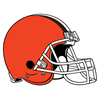BetMGM 2020 AFC Championship Odds
+290
2.9 to 1Ravens
21.3% implied probability

+290
2.9 to 1Chiefs
21.3% implied probability

+800
8 to 1Patriots
9.2% implied probability

+900
9 to 1Colts
8.3% implied probability

+1200
12 to 1Steelers
6.4% implied probability

+1400
14 to 1Bills
5.5% implied probability

+1400
14 to 1Chargers
5.5% implied probability

+2000
20 to 1Titans
4.0% implied probability

+2000
20 to 1Browns
4.0% implied probability

+2500
25 to 1Broncos
3.2% implied probability

No changes have been recorded yet.
+3000
30 to 1Texans
2.7% implied probability

+4000
40 to 1Raiders
2.0% implied probability

+4000
40 to 1Jets
2.0% implied probability

No changes have been recorded yet.
+5000
50 to 1Bengals
1.6% implied probability

+5000
50 to 1Dolphins
1.6% implied probability

+7000
70 to 1Jaguars
1.2% implied probability